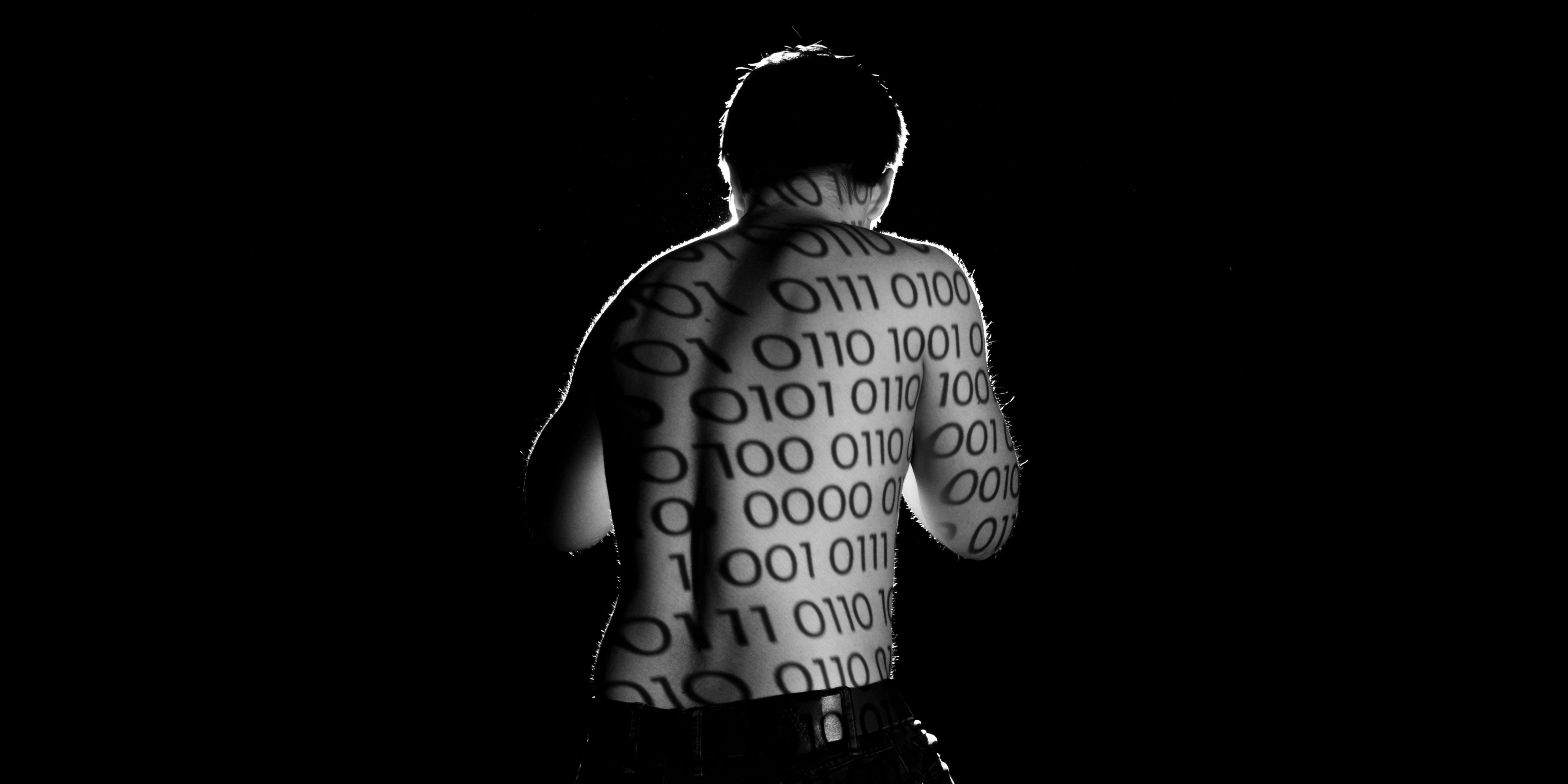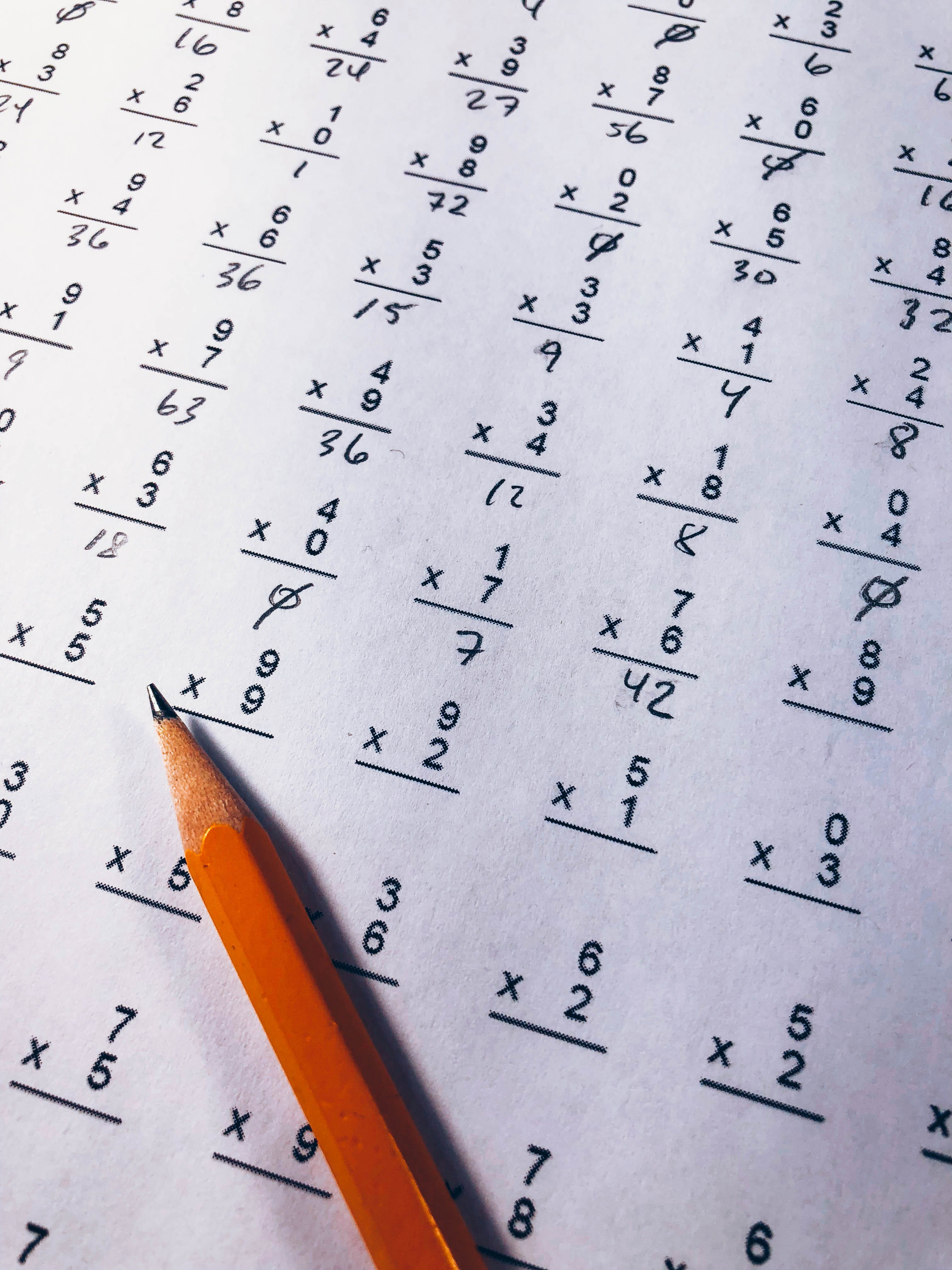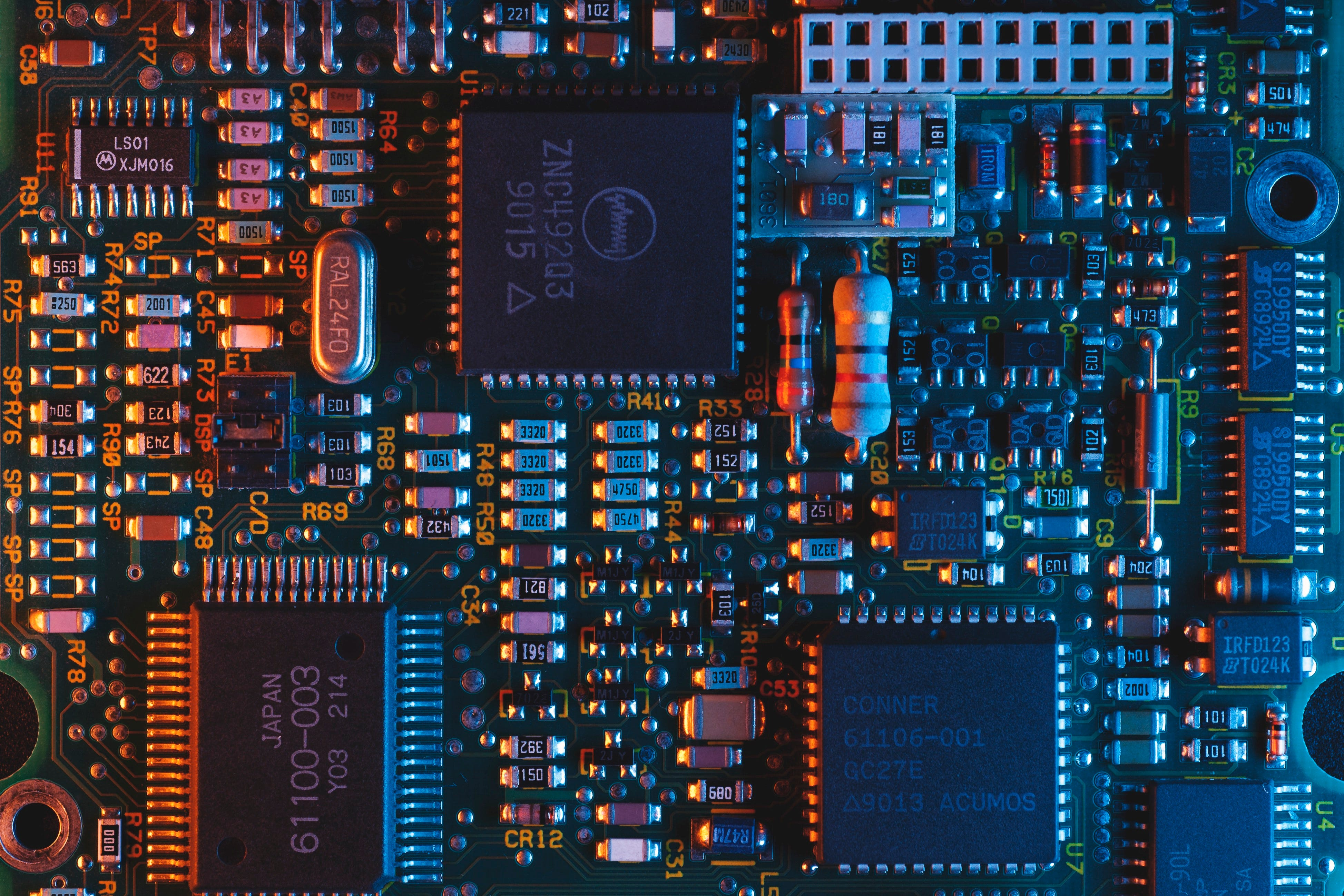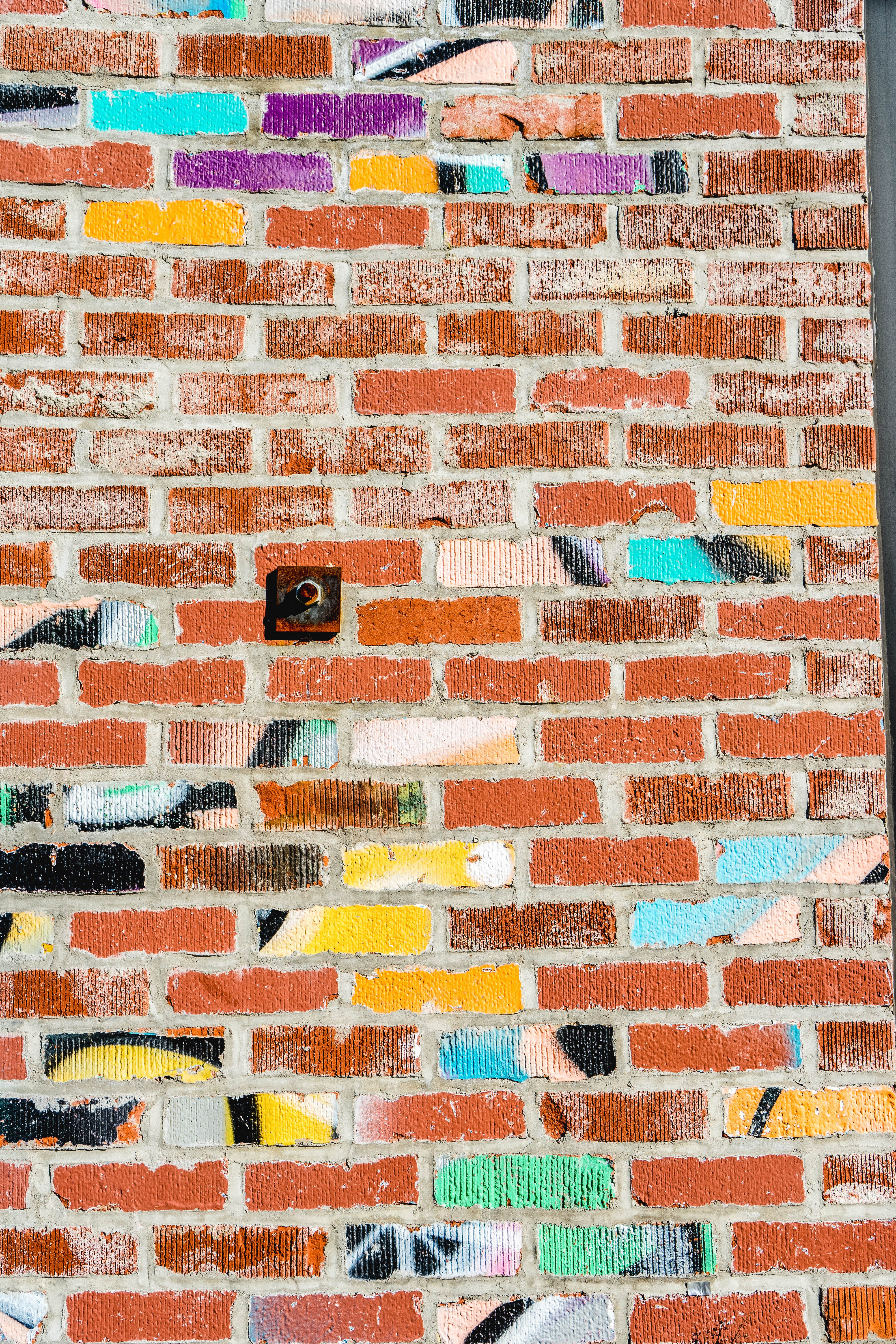# Understanding Binary

An Introduction to the 0s and 1sPhoto by Daniel Lanner on Unsplash

Even before I learned how to code, I knew there was something called binary and that it was the language computers spoke. I assumed that at some point during my 15 week bootcamp, we would discuss the elusive 0s and 1s but I was wrong. As it turns out, the code I was writing in JavaScript was being compiled and translated into 0s and 1s as instructions for the computer to understand. You may be thinking “ok, so I do not need to know about binary or number systems to code, then why should I bother learning it at all?” Even though understanding number systems is not required to code, it remains essential to our understanding of Computer Science. If you have any interest in taking a deep dive into how computers work, your starting point should be number systems and specifically, binary.Photo by Chris Liverani on Unsplash

Numbers are presented to us in something called place-value notation. This means that both the digit and its position(or place-value) must be known to determine its value.

Let’s look at this in the context of our number system. 5 by itself would be considered in the first position in our number system and therefore to determine its value we multiply it by 1 and get 5. What if the 5 is in the second position? The second positions in our number system multiplies the digit by 10, making its value 50. What if the 5 is in the third position? The third position multiplies the digit by 100, making its value 500.

I am sure most of you are saying “duh” right now. But breaking numbers down like this will help us understand other number systems we encounter. Another way of looking at the above is that each place value is determined by raising 10 to an ever increasing value (multiplying 10 by itself a certain number of times) and multiplying the result by the digit. For these reason, our number system is called base 10 or decimal. In the above examples:

5 in the first position = 5 *10⁰ = 5 * 1 = 5
5 in the second position = 5 * 10¹ = 5 * 10 = 50
5 in the third position = 5 * 10² = 5 * 100 = 500

The number 10 not only determines the order of magnitude for each place-value but also represents the number of possible values for each digit, which is 0–9. The above example only uses a single digit in different positions but what if we have multiple digits in different positions.

2 in the first position = 2*1⁰ = 2* 1 = 2
5 in the second position = 5 * 1¹ = 5 * 10 = 50
6 in the third position = 6* 1² = 6* 100 = 600
600 + 50 + 2 = 652

One thing to note is 0 which represents a position with no value, regardless of the position it is in. 0 times 10¹⁰ (10,000,000,000) is still 0. For this reason, the lowest digit that carries value is 1, not zero.

With only 10 possible digits in each position, what happens when the highest possible digit (9) increases by 1? We add the lowest digit that carries value to the next position and reset the current position to 0 or no value. In other words, 9 becomes 10.Photo by Umberto on Unsplash

Now that we have had a grade school math refresher and introduced some new glossary terms, we will break down binary in the same way we did our numeral system of base 10.

To begin, ask yourself what you know about binary. Odds are your answer will be that each position can only be a 0 or 1. Given what we discussed above, what base would binary be? The answer is 2. Base 2 not only means that each position can only have one of two possible digits but also that the order of magnitude of each place-value increases by powers of 2 instead of 10, as is the case with our number system.

Let us use the model from our base 10 examples to break down binary, using the number 1

1 at the first position (1) = 1 * 2⁰ = 1 * 1 = 1
1 at the second position (10) = 1 * 2¹ = 1 * 2 = 2
1 at the third position (100) =1 * 2² = 1 * 4 = 4
1 at the fourth position (1000) =1 * 2³ = 1 * 8 = 8
1 at the fifth position (10000) =1 * 2⁴ = 1 * 16 = 16

The above demonstrates how we can convert binary numbers to decimal based on 1 being at different positions and having different place-values. If the binary number is 11111, we can add all the above values up to get the total value in decimal

11111 = 1 + 10 + 100 + 1000 + 10000 = 1 + 2 + 4 + 8 + 16 = 31

Another way of looking at this is

11111 is 100000 minus 1 or
(1 * 2⁵) - 1 = 32 - 1 = 31

To make sense of this, we need to do some basic counting in binary. 0 and 1 are the only two possible numbers in each position with 1 being the number with the highest value. Starting with 0, if we add 1, we get 1 (same thing we see in decimal if we were to add 1 to 0). What happens if we add 1 to 1? Just as is the case with 9 in decimal, when we add 1 to 1 in binary, we add the lowest digit of value to the next position and reset the current position.

1 + 1 = 10

Following this pattern of increasing by 1

0
1
10
11
100
101
110
111
1000

Converting 0–100 in binary to decimal:

0= 0 * 2⁰ = 0 * 1 = 0
1= 1* 2⁰ = 1 * 1 = 1
10=(1*2¹) + (0 * 2⁰) = (1 * 2) + (0 * 1) = 2 + 0 = 2
11=(1* 2¹) + (1* 2⁰) = (1 * 2) + (1 * 1) = 2 + 1 = 3
100=(1*2²) + (0 * 2¹) + (0 * 2⁰)= (1 * 4) + (0 * 2) + (0 * 1) = 4 + 0 + 0 =4

This helps us understand binary as a number system but their true value in computers is more than simply representing decimal numbers in a different way.Photo by Tim Hüfner on Unsplash

How does a series of 0s and 1s become every computer application that has ever existed? Just as letters can be grouped to form words, words to form sentences, and sentences to form paragraphs. Binary can be grouped to represent everything from a simple decimal number (as we saw above) to complicated and elaborate instructions dictating the behavior of a character in a computer game.

Above, 0s and 1s were referred to as digits in the context of comparing binary to our number system. However, in the context of the code where binary is the foundation of every computer operation, 0s and 1s are bits. That is to say a 0 by itself is a bit and a 1 by itself is also a bit. When eight bits are grouped together, they form a byte. Finally a familiar term!

Besides numbers, one of the simplest uses of a byte is to represent a letter. This is done with the American Standard Code for Information Interchange, or ASCII, which “is a character encoding standard for electronic communication. ASCII codes represent text in computers”.

ABC: 01000001 01000010 01000011
Hello, world!: 01001000 01100101 01101100 01101100 01101111 00101100 00100000 01110111 01101111 01110010 01101100 01100100 00100001

(Make your own secret messages in binary here)

If one byte can only hold a single letter, you can imagine how many are needed for a song, movie, or game.

This is obviously and extensive topic but as the subtitle of this article says, this is meant to be an “Introduction to the 0s and 1s”, not a definitive guide. I hope this article can serve as a starting point for your own exploration into binary and other Computer Science concepts.

## Nerd For Tech

From Confusion to Clarification

## Nerd For Tech

NFT is an Educational Media House. Our mission is to bring the invaluable knowledge and experiences of experts from all over the world to the novice. To know more about us, visit https://www.nerdfortech.org/. Don’t forget to check out Ask-NFT, a mentorship ecosystem we’ve started

Written by

## Chase

Software Engineer // Coding, Laughing, TV, Movies, Art, Music, Food, Drink & Boston Terrier Enthusiast## Nerd For Tech

NFT is an Educational Media House. Our mission is to bring the invaluable knowledge and experiences of experts from all over the world to the novice. To know more about us, visit https://www.nerdfortech.org/. Don’t forget to check out Ask-NFT, a mentorship ecosystem we’ve started

Medium is an open platform where 170 million readers come to find insightful and dynamic thinking. Here, expert and undiscovered voices alike dive into the heart of any topic and bring new ideas to the surface. Learn more

Follow the writers, publications, and topics that matter to you, and you’ll see them on your homepage and in your inbox. Explore

If you have a story to tell, knowledge to share, or a perspective to offer — welcome home. It’s easy and free to post your thinking on any topic. Write on Medium